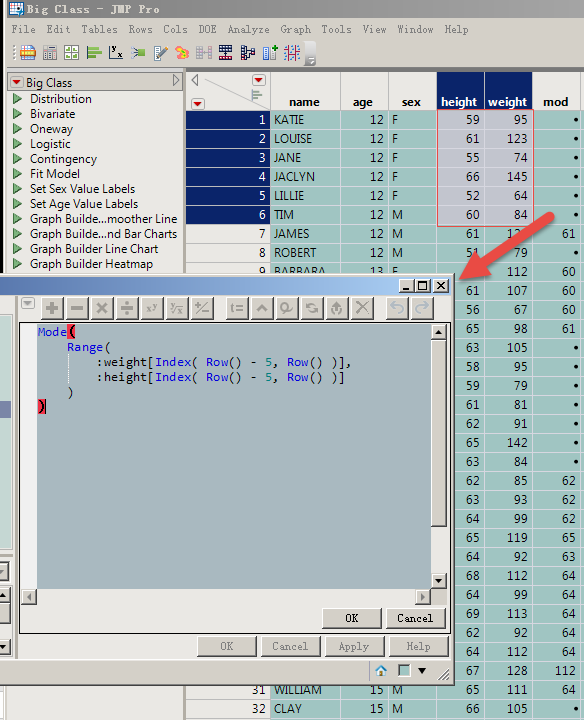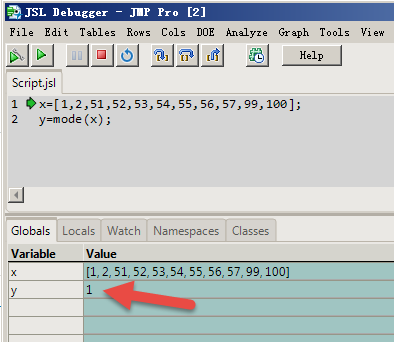Choose Language Hide Translation Bar
Highlighted

## How to find the mode of multiple columns and partial rows?

For example, add new columns with "Big Class. JMP ", and find the mode of the data in the same row of the "height"、"weight" columns and the five rows above.

Thanks!``mode(Range(:weight[Index(Row()-5,Row())], :height[Index(Row()-5,Row())]))``
1 ACCEPTED SOLUTION

Accepted Solutions
Highlighted

## Re: How to find the mode of multiple columns and partial rows?

Here is the formula that I came up with

``````If( Row() > 6,
Mode( Matrix( :height[Index( Row() - 5, Row() )] ) || Matrix( :weight[Index( Row() - 5, Row() )] ) ),
Mode( Matrix( :height[Index( 1, Row() )] ) || Matrix( :weight[Index( 1, Row() )] ) )
)``````

Note: The Range() function returns the minimum and maximum values found in the list or matrix

Note: JMP returns the minimum mode value if there is more than one mode

``mode([77,55,33,77,55])``

returns

`55`

while

``mode([55,34,67,88,65])``

returns

`34`

Jim
6 REPLIES 6
Highlighted

## Re: How to find the mode of multiple columns and partial rows?

• I can't get the right answer by using this formula.

Thanks!

Highlighted

## Re: How to find the mode of multiple columns and partial rows?

Here is the formula that I came up with

``````If( Row() > 6,
Mode( Matrix( :height[Index( Row() - 5, Row() )] ) || Matrix( :weight[Index( Row() - 5, Row() )] ) ),
Mode( Matrix( :height[Index( 1, Row() )] ) || Matrix( :weight[Index( 1, Row() )] ) )
)``````

Note: The Range() function returns the minimum and maximum values found in the list or matrix

Note: JMP returns the minimum mode value if there is more than one mode

``mode([77,55,33,77,55])``

returns

`55`

while

``mode([55,34,67,88,65])``

returns

`34`

Jim
Highlighted

## Re: How to find the mode of multiple columns and partial rows?

Thank Jim!

The JMP mode formula seems rather lax.

In Excel, no mode is returned as "#N/A", and JMP is returned as "minimum".

Highlighted

## Re: How to find the mode of multiple columns and partial rows?

The minimum is a correct mode.  There just happens to be more than one mode.

Jim
Highlighted

## Re: How to find the mode of multiple columns and partial rows?

Whether this can be verifiedHighlighted

## Re: How to find the mode of multiple columns and partial rows?

It is a simple matter to determine if more than one value was found that determined what the mode is.  If you want to set the resulting mode to a missing value when the mode comes from a single value, you can use the below logic

``````Names Default To Here( 1 );
mat = [1, 2, 3, 4, 5, 1];
xmode = Mode( mat );
If( N Rows( Loc( mat, xmode ) ) == 1,
x = .
);
Show( xmode );``````
Jim
Article Labels